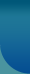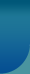Home >> Socio Short Notes >> Importance of Hypothesis

# Importance of Hypothesis

A hypothesis states what we are looking for. When facts are assembled, ordered and seen in a relationship they constitute a theory. The theory is not speculation but is built upon fact. Now the various facts in a theory may be logically analyzed and relationships other than those stated in the theory can be deduced. At this point there is no knowledge as to whether such deductions are correct. The formulation of the deduction however constitutes a hypothesis; if verified it becomes part of a future theoretical construction.

A hypothesis looks forward. It is a proposition which can be put to a test to determine its validity. It may seem contrary to or in accord with common sense. It may prove to be correct or incorrect. In any event however, it leads to an empirical test. Whatever the outcome, the hypothesis is a question put in such a way that an answer of some kind can be forthcoming. The function of the hypothesis is to state a specific relationship between phenomena in such a way that this relationship can be empirically tested. The basic method of this demonstration is to design the research so that logic will require the acceptance or rejection of the hypothesis on the basis of resulting data.© 2021 Sociology Guide.Com